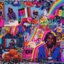Ask your own question, for FREE!
MathematicsOpenStudy (anonymous):

givne a matrix A m by n.....there exist matrices C and D such that such that cA = I of n by n and AD = I m by m prove that C=D and m =nOpenStudy (anonymous):

the problem is one of inverse matrices. given $AA^{-1} = A^{-1}A=I_x$ where x is the dimension of a square matrix, the rest should followOpenStudy (anonymous):

Suppose A is an m n matrix and there exist matrices C and D such that CA = In and AD = Im. Prove that m = n and C = D.OpenStudy (anonymous):

$X_{mn}Y_{kl}=Z_{ml}$ and $n=m$ for any matrices X,Y Since $I_n$ is a square matrix \A_{mn}C_{xy}=I_{n} \quad n=x,\text { and }m=y=n \]OpenStudy (anonymous):

$A_{mn}C_{xy}=I_{n} \quad n=x,\text { and }m=y=n$OpenStudy (anonymous):

sorry, and $n=k$

Can't find your answer? Make a FREE account and ask your own questions, OR help others and earn volunteer hours!

Join our real-time social learning platform and learn together with your friends!
Latest Questionsjmahoney: ya'll gonna do an update?
13 minutes ago 7 Replies 0 Medalskekeman: Did Gorbachev Implement policies to negatively restructure society and politics.
1 hour ago 0 Replies 0 MedalsNoodlesAndRiceYT: i think i found a glitch, sometimes when i log into questioncove, this happens an
1 hour ago 25 Replies 3 Medalsniahmk: What is the area of Triangle PQR on the grid? A triangle PQR is shown on a grid. The vertex P is on ordered pair 2 and 5, vertex Q is on ordered pair 4 and
9 minutes ago 1 Reply 0 Medalssnoopy007: (05.05 HC) A candy bar box is in the shape of a triangular prism. The volume of the box is 3,240 cubic centimeters.
2 hours ago 0 Replies 0 Medals14xavierrobinson: what is discrimination
3 hours ago 1 Reply 0 Medalsquasia: Who were the members of each alliance in World War I?
3 hours ago 5 Replies 1 MedalVocaloid: AP Calc BC Study Guide/Tutorial
3 hours ago 1 Reply 7 MedalsNoProbBob: Help Yo SS below
3 hours ago 15 Replies 1 Medalyallbabynene08: help
2 hours ago 20 Replies 2 Medals
Can't find your answer? Make a FREE account and ask your own questions, OR help others and earn volunteer hours!

Join our real-time social learning platform and learn together with your friends!